Saturday, July 11, 2020
Home > CBSE Class 12 > NCERT Solutions for Class 12 Maths Differential Equations Exercise 9.2

# NCERT Solutions for Class 12 Maths Differential Equations Exercise 9.2# NCERT Solutions for Class 12 Maths Differential Equations

Hi Students, Welcome to Amans Maths Blogs (AMB). In this post, you will get the NCERT Solutions for Class 12 Maths Differential Equations Exercise 9.2.

As we know that all the schools affiliated from CBSE follow the NCERT books for all subjects. You can check the CBSE NCERT Syllabus. Thus, NCERT Solutions helps the students to solve the exercise questions as given in NCERT Books.

NCERT Solutions for class 12 is highly recommended by the experienced teacher for students who are going to appear in CBSE Class 12 and JEE Mains and Advanced and NEET level exams. Here You will get NCERT Solutions for Class 12 Maths Differential Equations Exercise 9.2 of all questions given in NCERT textbooks of class 12 in details with step by step process.

The PDF books of NCERT Solutions for Class 12 are the first step towards the learning and understanding the each sections of Maths, Physics, Chemistry, Biology as it all help in engineering medical entrance exams. To solve it, you just need to click on download links of NCERT solutions for class 12.

CBSE Class 12th is an important school class in your life as you take some serious decision about your career. And out of all subjects, Maths is an important and core subjects. So CBSE NCERT Solutions for Class 12th Maths is major role in your exam preparation as it has detailed chapter wise solutions for all exercise. This NCERT Solutions can be downloaded in PDF file. The downloading link is given at last.

NCERT Solutions for Class 12 Maths are not only the solutions of Maths exercise but it builds your foundation of other important subjects. Getting knowledge of depth concept of CBSE Class 12th Maths like Algebra, Calculus, Trigonometry, Coordinate Geometry help you to understand the concept of Physics and Physical Chemistry.

## NCERT Solutions for Class 12 Maths Differential Equations

In each of the Exercises 1 to 10 verify that the given functions (explicit or implicit) is a solution of the corresponding differential equation.

NCERT Solutions for Class 12 Maths Differential Equations Exercise 9.2: Ques No 1.

Check whether the function y = ex + 1 is the solution of y″ – y′ = 0.

NCERT Solutions:

Given function is y = ex + 1         …(1)

Differentiating the equation (1) with respect to x, we getThus, the given function y = ex + 1 is the solution of y″ – y′ = 0.

NCERT Solutions for Class 12 Maths Differential Equations Exercise 9.2: Ques No 2.

Check whether the function y = x2 + 2x + C is the solution of y′ – 2x – 2 = 0.

NCERT Solutions:

Given function is y = x2 + 2x + C        …(1)

Differentiating the equation (1) with respect to x, we get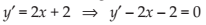Thus, the given function y = x2 + 2x + C is the solution of y′ – 2x – 2 = 0.

NCERT Solutions for Class 12 Maths Differential Equations Exercise 9.2: Ques No 3.

Check whether the function y = cos x + C is the solution of y′ + sin x = 0.

NCERT Solutions:

Given function is y = cos x + C        …(1)

Differentiating the equation (1) with respect to x, we get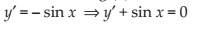Thus, the given function y = cos x + C is the solution of y′ + sin x = 0.

NCERT Solutions for Class 12 Maths Differential Equations Exercise 9.2: Ques No 4.

Check whether the function y = √(1 + x2) is the solution of y′ = xy/(1 + x2).

NCERT Solutions:

Given function is y = √(1 + x2)        …(1)

Differentiating the equation (1) with respect to x, we get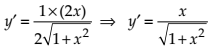…(2)

Dividing (2) by (1), we get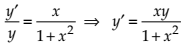Thus, the given function y = √(1 + x2) is the solution of y′ = xy/(1 + x2).

NCERT Solutions for Class 12 Maths Differential Equations Exercise 9.2: Ques No 5.

Check whether the function y = Ax is the solution of xy′ = y (x ≠ 0).

NCERT Solutions:

Given function is y = Ax        …(1)

Differentiating the equation (1) with respect to x, we get

y′ = A                                       …(2)

Dividing (2) by (1), we getThus, the given function y = Ax is the solution of xy′ = y (x ≠ 0).

NCERT Solutions for Class 12 Maths Differential Equations Exercise 9.2: Ques No 6.

Check whether the function y = x sin x is the solution of xy′ = y + x√(x2 – y2), (x ≠ 0 and x > y or x < – y).

NCERT Solutions:

Given function is y = x sin x        …(1)

Differentiating the equation (1) with respect to x, we get

y′ = x cos x + sin x                                   …(2)

Multiply x in (2), we get

xy′ = x2 cos x + x sin x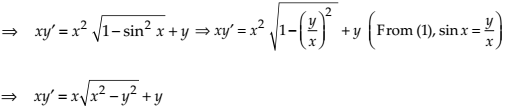Thus, the given function y = x sin x is the solution of xy′ = y + x√(x2 – y2), (x ≠ 0 and x > y or x < – y).

NCERT Solutions for Class 12 Maths Differential Equations Exercise 9.2: Ques No 7.

Check whether the function xy = log y + C is the solution of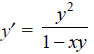, xy ≠ 1.

NCERT Solutions:

Given function is xy = log y + C        …(1)

Differentiating the equation (1) with respect to x, we get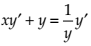…(2)Thus, the given function xy = log y + C is the solution of, xy ≠ 1.

NCERT Solutions for Class 12 Maths Differential Equations Exercise 9.2: Ques No 8.

Check whether the function y – cos y = x is the solution of (y sin y + cos y + x) y′ = y.

NCERT Solutions:

Given function is y – cos y = x        …(1)

Differentiating the equation (1) with respect to x, we get

y’ – (–sin y)y’ = 1 ⇒ y'(1 + sin y) = 1     …(2)

Multiply y in (2), we getThus, the given function y – cos y = x is the solution of (y sin y + cos y + x) y′ = y.

NCERT Solutions for Class 12 Maths Differential Equations Exercise 9.2: Ques No 9.

Check whether the function x + y = tan–1y is the solution of y2y′ + y2 + 1 = 0.

NCERT Solutions:

Given function is x + y = tan–1y        …(1)

Differentiating the equation (1) with respect to x, we get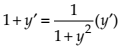Multiply y in (2), we getThus, the given function x + y = tan–1y is the solution of y2y′ + y2 + 1 = 0.

NCERT Solutions for Class 12 Maths Differential Equations Exercise 9.2: Ques No 10.

Check whether the functionis the solution of.

NCERT Solutions:

Given function is…(1)

Differentiating the equation (1) with respect to x, we getThus, the given functionis the solution of.

NCERT Solutions for Class 12 Maths Differential Equations Exercise 9.2: Ques No 11.

The number of arbitrary constants in the general solution of a differential equation of fourth order are:

(A) 0

(B) 2

(C) 3

(D) 4

NCERT Solutions:

(D)

Given function The number of arbitrary constant in the general solution of differential equation of nth order is n. So, the differential equation of fourth order have 4 constant.

NCERT Solutions for Class 12 Maths Differential Equations Exercise 9.2: Ques No 12.

The number of arbitrary constants in the particular solution of a differential equation of third order are:

(A) 3

(B) 2

(C) 1

(D) 0

NCERT Solutions:

(D)

error: Content is protected !!